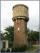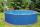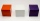# The pool

The cube-shaped pool has 140 cubic meters of water. Determine the dimensions of the bottom if the depth of the water is 200 cm and one dimension of the bottom is 3 m greater than the other. What are the dimensions of the pool bottom?

a =  10 m
b =  7 m

### Step-by-step explanation:

Our quadratic equation calculator calculates it.Did you find an error or inaccuracy? Feel free to write us. Thank you!Tips to related online calculators
Looking for help with calculating roots of a quadratic equation?
Do you know the volume and unit volume, and want to convert volume units?

#### You need to know the following knowledge to solve this word math problem:

We encourage you to watch this tutorial video on this math problem:

## Related math problems and questions:

• Swimming poolThe pool shape of a cuboid is 299 m3 full of water. Determine the dimensions of its bottom if the water depth is 282 cm and one bottom dimension is 4.7 m greater than the second.
• TrayWhat height reach water level in the tray shaped a cuboid, if it is 420 liters of water and bottom dimensions are 120 cm and 70 cm.
• Hexagonal prism 2The regular hexagonal prism has a surface of 140 cm2 and height of 5 cm. Calculate its volume.
• Swimming pool 4The pool shaped cuboid measuring 12.5 m × 640 cm at the bottom is 960hl water. To what height in meters reaches the water level?
• Water reservoirThe cuboid reservoir contains 1900 hectoliters of water and the water height is 2.5 m. Determine the dimensions of the bottom where one dimension is 3.2 m longer than the second one.
• RectangleThe length of the rectangle is 12 cm greater than 3 times its width. What dimensions and area this rectangle has if ts circumference is 104 cm.
• Cuboid - volume and areasThe cuboid has a volume of 250 cm3, a surface of 250 cm2, and one side 5 cm long. How do I calculate the remaining sides?
• 3rd dimensionThe block has a surface of 42 dm2 and its dimensions are 3 dm and 2 dm. What is the third dimension?
• Uboid volumeCalculate the cuboid volume if the walls are 30cm², 35cm², 42cm²
• Cuboid wallsCalculate the cuboid volume if its different walls have an area of 195cm², 135cm², and 117cm².
• Garden poolMr. Novak fill garden pool (cylinder; diameter of 200 cm) with 31.4 hl of water. What is the depth of the pool when the water level is 10 cm below the upper edge of the pool?
• The rainwaterThe rainwater container has the shape of a block whose bottom has dimensions of 4.5 m and 3.5 m. It is partly filled with water. What is the level if there is 189 hl of water in it?
• Water tankWhat is the height of the cuboid-shaped tank with the bottom dimensions of 80 cm and 50 cm if the 480 liters of water reaches 10 cm below the top?
• Cuboid and ratioFind the dimensions of a cuboid having a volume of 810 cm3 if the lengths of its edges coming from the same vertex are in ratio 2: 3: 5
• Water levelHow many cm will the water level in a cube-shaped tank with an edge of 3 m drop if we discharge 189 hl of water?
• Area and perimeter of rectangleThe content area of the rectangle is 3000 cm2, one dimension is 10 cm larger than the other. Determine the perimeter of the rectangle.
• The Scout TentThe Scout Tent has a rectangular wooden underlay with dimensions of 220 cm and 150 cm. How much canvas is needed for a 170 cm high pyramid roof?